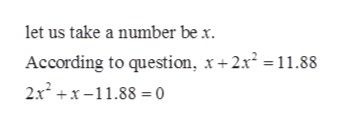# The sum of a positive number and twice its square is 11.88. What is the number.

Question
154 views

The sum of a positive number and twice its square is 11.88. What is the number.

check_circle

Step 1

Given, let us take a positive number behelp_outlineImage Transcriptioncloselet us take a number be x. According to question, x+2x2 11.88 2x2x-11.88 = 0 fullscreen
Step 2

### Want to see the full answer?

See Solution

#### Want to see this answer and more?

Solutions are written by subject experts who are available 24/7. Questions are typically answered within 1 hour.*

See Solution
*Response times may vary by subject and question.
Tagged in

### Polynomials